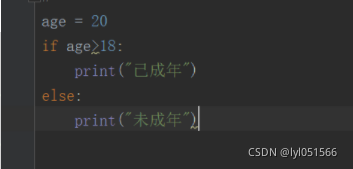﻿ Python的数据类型与标识符和判断语句详解_python_脚本之家
python# Python的数据类型与标识符和判断语句详解

## Python中的数据类型共有六个，分别是：

`字符串，数字，布尔类型，列表，元祖，字典，集合`

```可变类型：列表，字典，集合

### 1.列表和字典的区别：

```相同点：
1.都是可变类型
2.都是可迭代的

1.字典的key是不可变对象
2.字典的存储空间大于列表
3.字典的查询效率高于列表```

### 2.列表和元祖的区别：

```相同点：
1.都是可迭代的

1.列表可变，元组不可变
2.列表是动态的，长度大小不固定，可以进行增删改查
3.元组是静态的，长度在初始时已经确定不能更改```

### 3.列表和集合的区别：

```相同点：
1.都是可变类型

1.列表是有序的且元素不唯一，可以根据索引进行切片
2.集合是无序的且元素唯一
3.重复元素在集合中自动被过滤```

### 4.字典和集合的区别：

```相同点：
1.字典和集合都没有索引，不能进行切片和根据索引进行操作
2.都是可变类型

1.字典是键值对的形式
2.集合没有对应的value值```

## Python的常用标识符

```开发人员在程序中自定义的一些符号和名称

```1. 标识符只能由字母、下划线“_”、数字组成。
2. 标识符不能以数字开头。
3. 标识符不能使用关键字（不能与python中的关键字重名）。```

## Python中的关键字python一些具有特殊功能的标识符，这就是所谓的关键字

False        None       True       and        as      assert     async     await      break      class      continue      def      del      elif      else     except    finally     for   from         global         if          import        in         is  lambda     nonlocal      not      or      pass   raise return        try        while       with         yield

## 语句大纲### if判断语句

```if 要判断的条件:
条件成立时，要做的事情```

### 1.赋值情况下判断

```age = 20
if age>18:
print("已成年")
else:
print("未成年")```### 2.获取值的情况下判断

```age = input("请输入年龄：")
if int (age) >= 18:
print("已成年")
else:
print("未成年")```python中的比较运算符```a=10
b=20
print(a==b)
print("=======")
print(a!=b)
print("=======")
print(a>=b)
print("=======")
print(a<=b)
print("=======")
print(a>b)
print("=======")
print(a<b)```### 逻辑运算符### if-else### if嵌套```while 1:
age = input("请输入身高"),
if int(age)>150:
if int(age)<160:
print("半价进去吧")
else:
print("不免费")
else:
print("免费")```